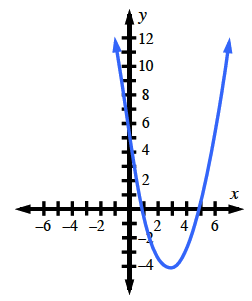### Home > INT2 > Chapter 12 > Lesson 12.1.4 > Problem12-52

12-52.
1. Sketch a graph of f(x) = x2 − 6x + 5. Homework Help ✎

1. Where is the vertex?

2. Describe the domain and range of this function.

3. Does the vertex represent the maximum or minimum value of the function?

4. For what values of x is the function positive? That is, for what values of x is f(x) > 0?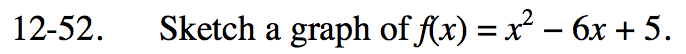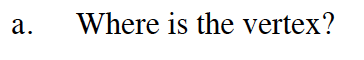Is the vertex the lowest or highest point on the graph?Remember that the domain is the set of all inputs for which there is an output, and the range is the set of all possible outputs.
Look at the graph. Does the graph extend all the way left and right (domain)?
Does the graph extend all the way up and down (range)?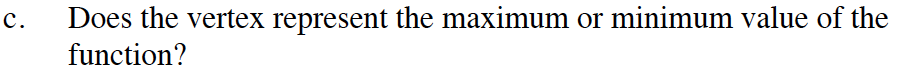The vertex is the lowest/highest point on the graph.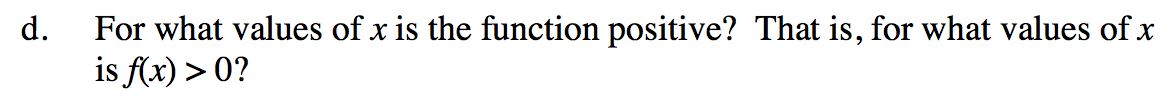x ≤ 1; x ≥ 5